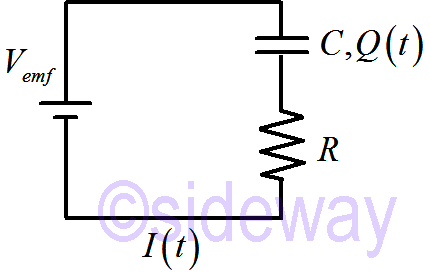output.to from Sideway
Draft for Information Only

# Content

`Time Constant of RC Circuit Current in RC Circuit Source and Reference`

# Time Constant of RC CircuitImmediately after the RC circuit is connected to battery, `𝑄=0; 𝑉𝑒𝑚𝑓=𝐼𝑅` After the capacitor is fully charged, `𝑄=𝐶𝑉𝑒𝑚𝑓; 𝐼=0`

## Current in RC Circuit

The current in RC Circuit is a function of time. Current is defined as Charge ∆𝑄 passed by per unit time ∆𝑡 ```𝐼=|𝑞|𝑛𝐴𝑣⇒𝐼=∆𝑄∆𝑡 lim ∆𝑡→0⇒𝐼=𝑑𝑄𝑑𝑡``` Since current 𝐼 is the same everywhere in the RC circuit, charge ∆𝑄 passed by per unit time ∆𝑡 throughout the circuit piles up at the capacitor 𝐶. The voltage 𝑉𝐶 across the capacitor is 𝑄𝐶 and voltage 𝑉𝑅 across the resistor is 𝐼𝑅. Apply voltage loop ```𝑉𝑒𝑚𝑓=𝑉𝐶+𝑉𝑅=𝑄𝐶+𝐼𝑅=𝑄𝐶+𝑑𝑄𝑑𝑡𝑅 ⇒𝑑𝑄(𝑡)𝑑𝑡=𝑉𝑒𝑚𝑓𝑅−1𝑅𝐶𝑄(𝑡) ``` Assume 𝑄(𝑡) has the form 𝐴𝑒−𝑡/𝑅𝐶+constant, ```𝑄(𝑡)=𝐴𝑒−𝑡/𝑅𝐶+constant⇒𝑑𝑄(𝑡)𝑑𝑡=−1𝑅𝐶𝐴𝑒−𝑡/𝑅𝐶=−1𝑅𝐶𝑄(𝑡)−constant  =1𝑅𝐶constant−1𝑅𝐶𝑄(𝑡)=𝑉𝑒𝑚𝑓𝑅−1𝑅𝐶𝑄(𝑡) ⇒constant=𝑉𝑒𝑚𝑓𝐶⇒𝑄(𝑡)=𝐴𝑒−𝑡/𝑅𝐶+𝑉𝑒𝑚𝑓𝐶 ``` Apply boundary conditions, At 𝑡=0, 𝑄=0 `𝑄(𝑡→0)=𝐴𝑒−0/𝑅𝐶+𝑉𝑒𝑚𝑓𝐶=𝐴+𝑉𝑒𝑚𝑓𝐶=0⇒𝐴=−𝑉𝑒𝑚𝑓𝐶` Therefore ```𝑄(𝑡)=(−𝑉𝑒𝑚𝑓𝐶)𝑒−𝑡/𝑅𝐶+𝑉𝑒𝑚𝑓𝐶=𝑉𝑒𝑚𝑓𝐶1−𝑒−𝑡/𝑅𝐶 I(𝑡)=𝑑𝑄𝑑𝑡=−𝑉𝑒𝑚𝑓𝐶−1𝑅𝐶𝑒−𝑡/𝑅𝐶=𝑉𝑒𝑚𝑓𝑅𝑒−𝑡/𝑅𝐶 ``` 𝑅𝐶 is the time constant of a RC circuit. When time 𝑡=𝑅𝐶, the current 𝐼 drops by a factor of 𝑒. `I(𝑡)=𝑉𝑒𝑚𝑓𝑅𝑒−𝑡/𝑅𝐶=𝑉𝑒𝑚𝑓𝑅𝑒−1=12.718𝑉𝑒𝑚𝑓𝑅=0.37𝑉𝑒𝑚𝑓𝑅` By Talor Expansion `𝑒−𝑡/𝑅𝐶=1−𝑡𝑅𝐶+𝑡𝑅𝐶212−⋯` When time 𝑡 and 𝑅𝐶 are equal, the coefficients of Taylor Expansion equal to 1. In other words, at the time constant 𝑅𝐶 the current is always reduced to 0.37 of the original current.

## Source and Reference

ID: 200100102 Last Updated: 1/1/2020 Revision: 0Home 5

Management

HBR 3

Information

Recreation

Culture

Chinese 1097

English 337

Computer

Hardware 156

Software

Application 207

Latex 35

Manim 203

Numeric 19

Programming

Web 285

Unicode 504

HTML 65

CSS 65SVG 9

ASP.NET 270OS 422

Python 66Knowledge

Mathematics

Algebra 84

Geometry 32

Calculus 67

Engineering

Mechanical

Rigid Bodies

Statics 92

Dynamics 37

Control

Natural Sciences

Electric 27